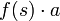# Action of wreath product on Cartesian product

Suppose both$G$ and$H$ are being viewed along with specific group actions of each, i.e., they are both being viewed as groups of permutations, with$G$ acting on a set$A$ and$H$ acting on a set$S$. Then,$G \wr H$ comes equipped with a natural action on the Cartesian product$A \times S$ as follows.
First, recall that$G \wr S$ is the external semidirect product$G^S \rtimes H$. To specify an action of this, we will specify how$G^S$ and$H$ act; then, via the equivalence of internal and external semidirect product, we would have defined the action of a generating set and hence of all of$G \wr H$. Here is the action:
•$G^S$ acts on$A \times S$ as follows: An element of$G^S$ is a function$f:S \to G$. For an element$(a,s)$, the action of$f$ on$(a,s)$ is obtained as$(f(s) \cdot a, s)$ where$f(s) \cdot a$ is the action of the element$f(s) \in G$ on$A$. In other words, it permutes each of the fibers according to the$f$-value on that fiber.
•$H$ acts on$A \times S$ as follows: An element$h \in H$ sends$(a,s) \in A \times S$ to$(a,h \cdot s)$ where$h \cdot s$ denotes the image of$s$ under the action of$h$.